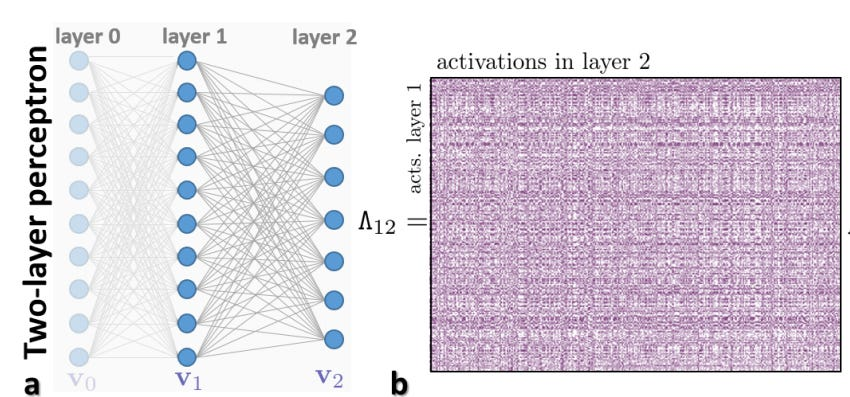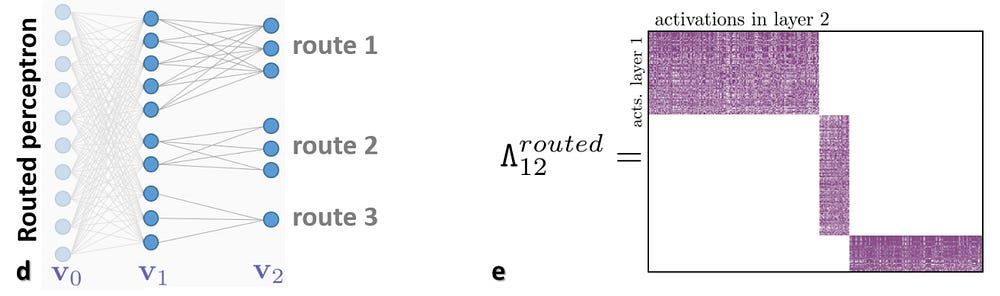Conditional Neural Networks

Hello! This is my analysis of the paper Decision Forests, Convolutional Networks and the Models in-Between by Ioannou et. al. which came from Microsoft Research. In the following discussion, I give an explanation of the intricacies of the model as well as provide some details on the implementation. As the title of the paper suggests, the paper presents a continuum of models which are hybrids of Decision Forests and Neural Networks and the authors call them, Conditional Neural Networks. As we know, Decision forests are computationally efficient thanks to their conditional computation property (computation is confined to only a small region of the tree, the nodes along a single branch). While on the other end of the spectrum, CNNs achieve state of the art accuracy, thanks to their representation learning capabilities. The continuous set of models presented in the paper lie in between the two extremes and we can tune the hyper-parameters to generate the model of our will, whether we want it to be more efficient or we want it to be more accurate.

General Intuition

In this section, I discuss the intuition behind the model. So, what the authors did was, they trained the VGG Model on the Image-Net data-set and tried to observe the co-relation between the activations of consecutive layers. For starters, lets observe the co-relation between the activations of two consecutive layers in a part of the VGG Model.On the left is the said model, and on the right is the co-relation matrix between the activations of the last two layers.

Looking at the above matrix, it appears that there is no structure to it and the co-relation matrix seems like random noise. However, if we rearrange the rows and columns, we will see the structure that is hidden beneath.

The above image reveals an underlying block-diagonal structure to the model. Now, what does a block-diagonal structure mean? It means that the activation of a bunch of neurons in the later layer is highly co-related to that of another bunch in the previous layer, whereas it does not depend much on the activations of other neurons. Now, if the activation of a neuron does not affect the activation or non-activation of another neuron in the next layer, it is really futile to connect the two. So, this observation leads to the beginning of a tree-like structure, if we disconnect the connections between neurons with low co-relation coefficients, as shown below.On the left is the model obtained after disconnecting the said neurons and on the right is the co-relation matrix for this model. Note that the neurons which had low co-relation coefficients in the original model have now zero co-relation coefficient because they have been completely disconnected.

The above observations are indicative of the fact that we can make deep models in which there need not be fully-connected layers, instead of which, we can have block-wise connected layers which can be modelled into a decision-tree like framework, to which we will come later. For now, lets just move forward, keeping in mind that such block-wise connected models will have similar performance accuracy vise and should be expected to be more efficient, since the decrease in number of connections also results in decrease in number of parameters. In fact, you can easily derive a general expression for the number of parameters in the newer model as compared to the previous one. I will not derive it here and will instead, move on to the next part.

Neural Networks and Decision Trees are actually quite similar

Let’s introduce a new notation for both Neural Networks as well as for Decision Forests which will allow us to look at both models with same framework. Going further, I will describe Conditional Neural Networks using the same notation.

Neural Networks

What Neural Networks actually do is that, our data undergoes several non-linear transformations and by the time it reaches the final layer, it is transformed enough that it can be easily separated. This is the basis of Neural Networks in over-simplified terms. Now, imagine each layer doing the above mentioned task of first linearly transforming a data, by multiplying it with a matrix and then applying a non-linear function on it, which in our case is the ReLU function. The symbol P_ij | denotes the popular nonlinear transformation v_j = σ(Pv_i) between two consecutive layers i and j. The linear projection matrix is denoted ‘P’, and ‘|’ indicates a non-linear function σ.

Decision Trees

Since in Decision Trees, the data flows untransformed, the linear transform function will be nothing but an Identity Matrix and we won’t apply the non-linear transform. Another striking difference here would be the presence of explicit routers. Since the data in Decision Forests doesn’t flow into all of it’s children we will have to incorporate routers into our model. We can do this by having a block of size equal to the number of children and apply softmax to it. Now, we can choose top k probabilities and send the data to the corresponding k children. For conventional Decision Tress, k is usually equal to one. We can also easily extend this model to stochastic forests by just multiplying the output of the router block with the output of the layer and in that way we will have decision forests with stochastic routing.

Conditional Neural Networks

Now that we have seen Neural Networks and Decision Trees from a joint point of view, it is really easy to introduce CondNNs. We can think of them in two ways. One is decision trees augmented with data transformation operators and the other one is CNNs, with block-diagonal sparse weight matrices, and explicit data routing functions. Let us first focus on the first way of visualisation. CondNNs are just Decision Trees, but instead of passing forward the data as it is, each node applies a non-linear transformation to the data. And, with the help of routers, we can forward the data to one or more of its children. The other point-of-view is just that we see the whole model as a neural network and instead of having fully connected layers, we have block-vise connected layers. And that’s pretty much it.